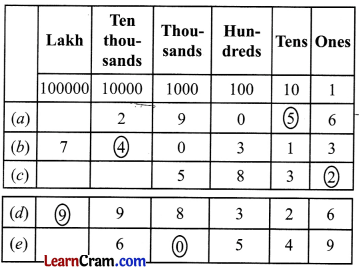# DAV Class 4 Maths Chapter 1 Worksheet 4 Solutions

The DAV Class 4 Maths Book Solutions and DAV Class 4 Maths Chapter 1 Worksheet 4 Solutions of Numbers up to 999999 offer comprehensive answers to textbook questions.

## DAV Class 4 Maths Ch 1 WS 4 Solutions

Question 1.
Look at the place value chart given below and write the place of the encircled digits.(a) Place value of 5 is 5 × 10 or fifty.
(b) Place value of 4 is 4 × 10000 or forty thousand.
(c) Place value of 2 is 2 × 1 or two.
(d) Place value of 9 is 9 × 100000 or 9 lakh.
(e) Place value of 0 is 0 × 1000 or 0.

Question 2.
Write down the place value of the digit in bold.
(a) 3491
(b) 80102
(c) 321893
(d) 6505
(e) 76321
(f) 19568
(g) 235740
(h) 4923
(a) Place value of 4 is 4 × 100 or 4 hundreds.
(b) Place value of 0 is 0 × 1000 or 0.
(c) Place value of 3 is 3 × 100000 or 3 lakhs.
(d) Place value of 0 is 0 × 10 or 0.
(e) Place value of 6 is 6 × 1000 or 6 thousands.
(f) Place value of 8 is 8 × 1 or 8.
(g) Place value of 2 is 2 × 100000 or 2 lakhs.
(h) Place value of 9 is 9 × 100 or 9 hundreds.Question 3.
Underline the numeral in which the place value of 8 is 80000.
(a) 38291
(b) 4328
(c) 84720
(d) 829
(c) 84720.

Question 4.
Underline the numeral in which the place value of 2 is 200.
(a) 253410
(b) 48295
(c) 72843
(d) 45782